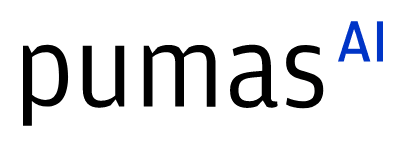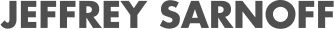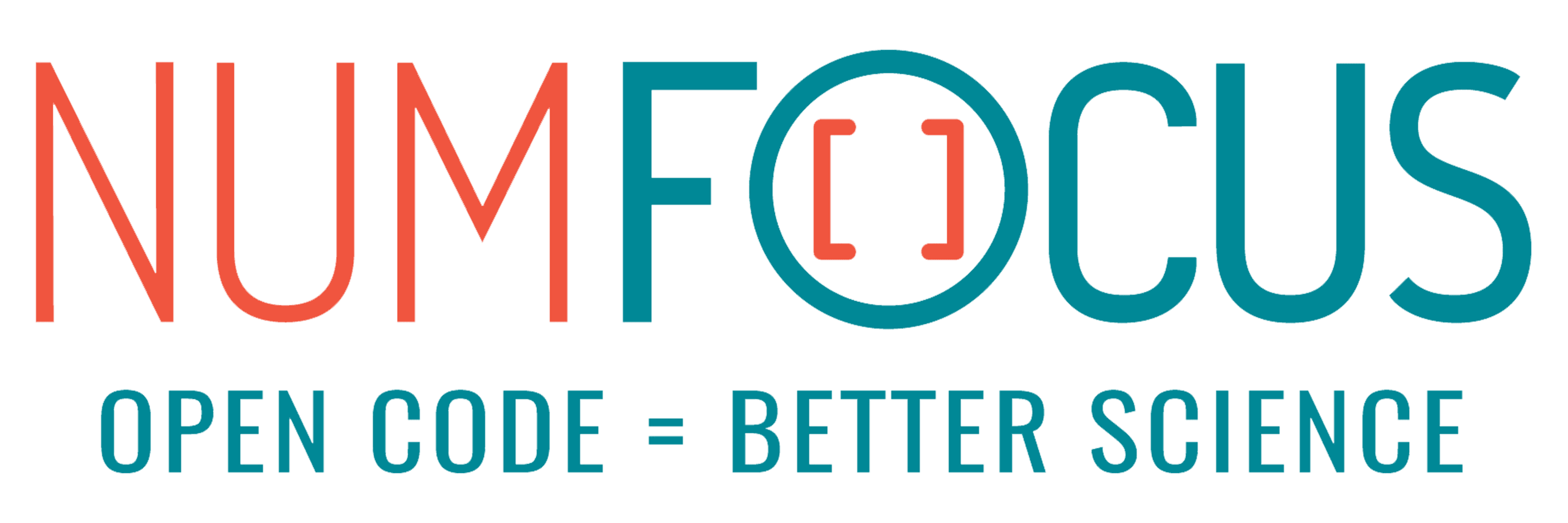# Cycles and Julia Sets: Novel algorithms for Numerical Analysis

07/27/2022, 3:10 PM3:20 PM UTC
Purple

#### Abstract:

We present a new collection of algorithms dedicated to compute the basins of attraction of any complex rational map. This is a relevant matter in Holomorphic Dynamics, and also a way to visualize and study amazing fractal objects like Julia Sets. These algorithms solve many computational problems that often arise in Numerical Analysis, like overflows or mathematical indeterminations, and provide more information about the dynamics of the system than traditional algorithms generally do.

#### Description:

In this talk we will present a new collection of algorithms dedicated to compute the basins of attraction of any complex rational map, and to study the dynamical behaviour of its fixed points and attracting n-cycles. By doing this, one can visualize and study amazingly beautiful and complex fractal objects like Julia Sets. The study of the basins of attraction of a dynamical system is a very relevant matter not only in Numerical Analysis and Holomorphic Dynamics, but also in other fields like Physics or Mechanical Engineering.

We are going to describe the methods implemented in the Lyapunov Cycle Detector module, available in the following GitHub repository: https://github.com/valvarezapa/LCD. In it you will find the code itself, several explanations of how does the methods work, what do they do, a lot of practical examples and even a User Guide.

Our work is based in very relevant theorems of Complex Dynamics, like the Ergodic Theorem, and is motivated by Sullivan's work on Dynamical Systems, recently awarded with the Abel prize. However, no previous knowledge about any of these topics is required, since we will focus on the algorithms and the implementation of the code. The graphics we will be able to generate are both rich and beautiful, and the concepts behind them are easy to grasp. Everyone interested in the mathematical framework or in any specific technicalities behind these algorithms can consult our paper "Algorithms for computing attraction basins of a self-map of the Hopf fibration based on Lyapunov functions" (currently on preprint).

From a scientific computing point of view, this new collection of algorithms solve most of the computational problems that often arise in Numerical Analysis, like overflows or mathematical indeterminations. We achieve this by considering the Hopf endomorphism induced by the given rational map, and iterating it over the complex projective line (P^1(C)). This approach also allows us to easily work with the infinity point. Since this kind of calculations often have high computational cost, we benefit from Julia’s efficiency and some built-in multi-threading macros in order to be able to visualize the results in a reasonable amount of time.

From a mathematical perspective, we will be considering a discrete-time dynamical system given by a complex rational map. The techniques we use to compute the basins of attraction are based on Lyapunov functions and Lyapunov coefficients (which are closely related to Lyapunov exponents; a very powerful and commonly used concept in dynamical systems). The Lyapunov function we define is constant in each basin of attraction, and depends on the notion of spherical derivative of the given rational map. This way, we can divide the Riemann Sphere (the plane of complex numbers adding the infinity point) into the different basins of attraction (each one with an associated constant) and the Julia set. Most famous Julia sets can be computed and visualized this way. Also, our algorithms provide more information about the dynamics of the system than most traditional algorithms on this topic generally do. Our methods are focused on detecting the attracting n-cycles of the given rational map and its basins. Note that fixed points are just the particular case of 1-cycles. In addition, by using Lyapunov coefficients we are able to measure how much attracting is every n-cycle.## Media partners## Community partners